Next: Waveshaping Up: Modulation Previous: Taxonomy of spectra   Contents   Index

# Multiplying audio signals

We have been routinely adding audio signals together, and multiplying them by slowly-varying signals (used, for example, as amplitude envelopes) since Chapter 1. For a full understanding of the algebra of audio signals we must also consider the situation where two audio signals, neither of which may be assumed to change slowly, are multiplied. The key to understanding what happens is the Cosine Product Formula:To see why this formula holds, we can use the formula for the cosine of a sum of two angles:to evaluate the right hand side of the cosine product formula; it then simplifies to the left hand side.

We can use this formula to see what happens when we multiply two sinusoids (Page):In words, multiply two sinusoids and you get a result with two partials, one at the sum of the two original frequencies, and one at their difference. (If the differencehappens to be negative, simply switch the original two sinusoids and the difference will then be positive.) These two new components are called sidebands.

This gives us a technique for shifting the component frequencies of a sound, called ring modulation, which is shown in its simplest form in Figure 5.2. An oscillator provides a carrier signal, which is simply multiplied by the input. In this context the input is called the modulating signal. The term ring modulation" is often used more generally to mean multiplying any two signals together, but here we'll just consider using a sinusoidal carrier signal. (The technique of ring modulation dates from the analog era [Str95]; digital multipliers now replace both the VCA (Section 1.5) and the ring modulator.)Figure 5.3 shows a variety of results that may be obtained by multiplying a (modulating) sinusoid of angular frequencyand peak amplitude, by a (carrier) sinusoid of angular frequency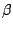and peak amplitude 1:(For simplicity the phase terms are omitted.) Each part of the figure shows both the modulation signal and the result in the same spectrum. The modulating signal appears as a single frequency,, at amplitude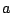. The product in general has two component frequencies, each at an amplitude of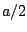.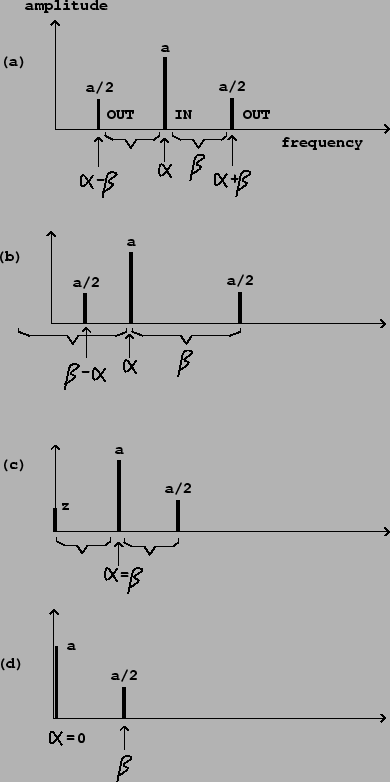Parts (a) and (b) of the figure show general" cases whereandare nonzero and different from each other. The component frequencies of the output are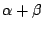and. In part (b), since, we get a negative frequency component. Since cosine is an even function, we haveso the negative component is exactly equivalent to one at the positive frequency, at the same amplitude.

In the special case where, the second (difference) sideband has zero frequency. In this case phase will be significant so we rewrite the product with explicit phases, replacingby, to get:The second term has zero frequency; its amplitude depends on the relative phase of the two sinusoids and ranges from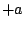toas the phase differencevaries fromto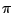radians. This situation is shown in part (c) of Figure 5.3.

Finally, part (d) shows a carrier signal whose frequency is zero. Its value is the constant(not; zero frequency is a special case). Here we get only one sideband, of amplitudeas usual.

We can use the distributive rule for multiplication to find out what happens when we multiply signals together which consist of more than one partial each. For example, in the situation above we can replace the signal of frequencywith a sum of several sinusoids, such as:Multiplying by the signal of frequencygives partials at frequencies equal to: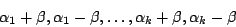As before if any frequency is negative we take its absolute value.

Figure 5.4 shows the result of multiplying a complex periodic signal (with several components tuned in the ratio 0:1:2:) by a sinusoid. Both the spectral envelope and the component frequencies of the result are changed according to relatively simple rules.The resulting spectrum is essentially the original spectrum combined with its reflection about the vertical axis. This combined spectrum is then shifted to the right by the carrier frequency. Finally, if any components of the shifted spectrum are still left of the vertical axis, they are reflected about it to make positive frequencies again.

In part (b) of the figure, the carrier frequency (the frequency of the sinusoid) is below the fundamental frequency of the complex signal. In this case the shifting is by a relatively small distance, so that re-folding the spectrum at the end almost places the two halves on top of each other. The result is a spectral envelope roughly the same as the original (although half as high) and a spectrum twice as dense.

A special case, not shown, is to use a carrier frequency half the fundamental. In this case, pairs of partials will fall on top of each other, and will have the ratios 1/2 : 3/2 : 5/2 :to give an odd-partial-only signal an octave below the original. This is a very simple and effective octave divider for a harmonic signal, assuming you know or can find its fundamental frequency. If you want even partials as well as odd ones (for the octave-down signal), simply mix the original signal with the modulated one.

Part (c) of the figure shows the effect of using a modulating frequency much higher than the fundamental frequency of the complex signal. Here the unfolding effect is much more clearly visible (only one partial, the leftmost one, had to be reflected to make its frequency positive). The spectral envelope is now widely displaced from the original; this displacement is often a more strongly audible effect than the relocation of partials.

As another special case, the carrier frequency may be a multiple of the fundamental of the complex periodic signal; then the partials all land back on other partials of the same fundamental, and the only effect is the shift in spectral envelope.Next: Waveshaping Up: Modulation Previous: Taxonomy of spectra   Contents   Index
Miller Puckette 2006-12-30